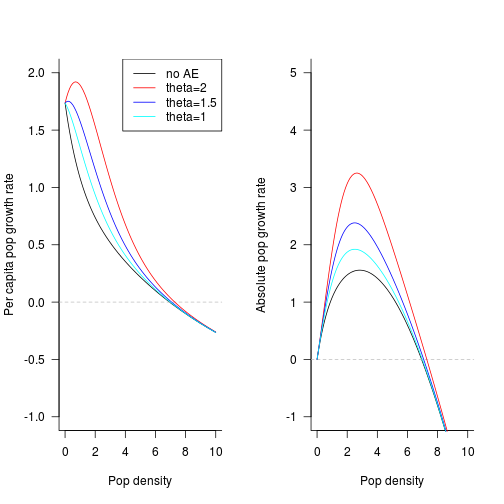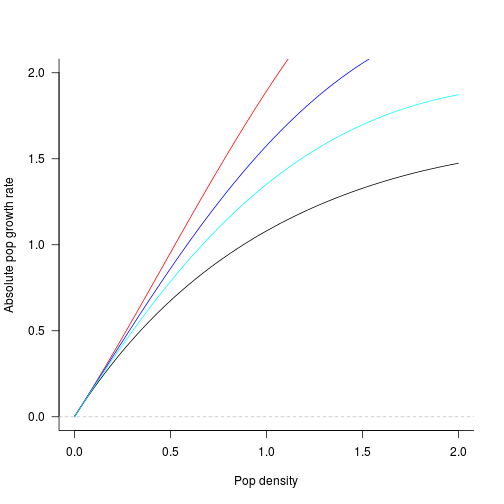## weak vs strong Allee effects

An Allee effect means that the per capita growth rate increases rather than decreases with population density over some range (typically for small populations). A strong Allee effect means that the per capita growth rate is negative when density is zero (or in the limit as density goes to zero); a weak Allee effect means the growth rate is positive near zero.

Because the AE is defined in terms of the per capita growth rate, it's easy to detect one by looking at a plot of per capita growth rate vs. density; if the growth rate is ever increasing, then there's an AE (and vice versa). It's a little bit harder to tell from looking at per capita birth and death plotted together (unless one of them is constant), because you have to figure out whether the birth rate is increasing faster than the death rate.

Strong Allee effects are easy to detect because the absolute growth rate as well as the per capita growth rate must be negative near $$N=0$$ if there is strong AE (and vice versa).

How do we detect a weak AE? Define $$r(N)$$ as the per capita growth rate at density $$N$$, $$r'(N)$$ as $$d(r(N))/dN$$, $$r''(N)$$ as $$d^2(r(N))/dN^2$$. Then we want to know what conditions on the absolute growth rate $$N r(N)$$ as a function of $$N$$ imply an AE of any kind (including weak AEs), and vice versa.

The derivative of the absolute growth rate is $\frac{d(N r(N)}{dN} = r(N) + N r'(N)$ so the absolute growth rate will actually decrease initially if $$r(0)<0$$ (i.e. a strong AE).

The second derivative of the absolute growth rate is $\frac{d^2(N r(N)}{dN^2} = r'(N) + r'(N) + N r''(N) = 2 r'(N) + N r''(N)$

If we let $$N>0$$ then this is hairy – we need to know the relationship between $$r''(N)$$ and $$r'(N)$$ – but in the limit as $$N \to 0$$ (actually we don't really need the limit …) it reduces to $$2 r'(0)$$, which means there will be an AE iff the absolute growth rate has a positive second derivative, i.e. it is initially accelerating.

It is technically with the definition of an AE to have $$r'(0)$$ negative but $$r'(N)$$ positive for some non-zero range of $$N$$, but that's a pretty weird case, I'm going to ignore it.

Define per capita growth rate function: $r(N) = b_0 \left( (x+1)^\theta \exp(-(x+1)/a + \exp(-x/b_s)) \right) - d$ the $$x+1$$ in the AE part of the equation is a bit of a hack – the behaviour of the power function for $$0 < x < 1$$ is not what I want, so I avoided it. (It could also be due to the original sin of raising a unitful quantity to a power?)

afun_pc <- function(x, d = 1, b0 = 2, bscale = 10, aepow = 0, aescale = 1) {
b0 * ((x + 1)^aepow * exp(-(x + 1)/aescale) + exp(-x/bscale)) - d
}
afun_abs <- function(x, ...) {
x * afun_pc(x, ...)
}


I have to work out the derivative, it's a little hairier than I thought. Ideally I would like to plot this for $$b_s \gg a \gg 1$$, so that all but the $$(x+1)^\theta$$ terms drop out near zero …

par(las = 1, bty = "l", mfrow = c(1, 2))
curve(afun_pc, from = 0, to = 10, ylim = c(-1, 2), xlab = "Pop density", ylab = "Per capita pop growth rate")
abline(h = 0, lty = 2, col = "gray")
curve(afun_pc(x, aepow = 2), add = TRUE, col = 2)
curve(afun_pc(x, aepow = 1.5), add = TRUE, col = 4)
curve(afun_pc(x, aepow = 1), add = TRUE, col = 5)
legend("topright", col = c(1, 2, 4, 5), legend = c("no AE", "theta=2", "theta=1.5",
"theta=1"), lty = 1)
curve(afun_abs, from = 0, to = 10, ylim = c(-1, 5), xlab = "Pop density", ylab = "Absolute pop growth rate")
abline(h = 0, lty = 2, col = "gray")
curve(afun_abs(x, aepow = 2), add = TRUE, col = 2)
curve(afun_abs(x, aepow = 1.5), add = TRUE, col = 4)
curve(afun_abs(x, aepow = 1), add = TRUE, col = 5)Just by eye, it looks like $$\theta>1$$ (maybe even bigger) is necessary for an AE with these parameters.

It's awfully hard to see that these curves have positive curvature, even when we zoom in a bit:

par(las = 1, bty = "l")
curve(afun_abs, from = 0, to = 2, ylim = c(0, 2), xlab = "Pop density", ylab = "Absolute pop growth rate")
abline(h = 0, lty = 2, col = "gray")
curve(afun_abs(x, aepow = 2), add = TRUE, col = 2)
curve(afun_abs(x, aepow = 1.5), add = TRUE, col = 4)
curve(afun_abs(x, aepow = 1), add = TRUE, col = 5)